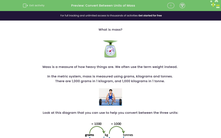# Convert Between Units of Mass

In this worksheet, students will convert between units of mass.Key stage:  KS 3

Curriculum topic:   Number

Curriculum subtopic:   Use Standard Units of Measure

Difficulty level:#### Worksheet Overview

What is mass?Mass is a measure of how heavy things are. We often use the term weight instead.

In the metric system, mass is measured using grams, kilograms and tonnes.

There are 1,000 grams in 1 kilogram, and 1,000 kilograms in 1 tonne.Look at this diagram that you can use to help you convert between the three units:If we have a quantity measured in kilograms and we want to change it to grams, how do we do this?

We want the arrow pointing from kg to g so we multiply by 1,000.

Example:

Change 56 kg into g:

56 x 1,000 = 56,000

So 56 kg = 56,000 g

And if we want to change a quantity from kg to tonnes? We're going the opposite way, so we divide by 1,000.

Example:

Change 450 kg into tonnes

450 ÷ 1,000 = 0.45

So 450 kg = 0.45 t